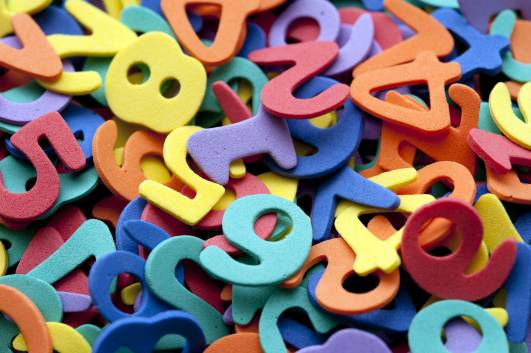# What is 659.1649 rounded to the nearest tenth?

659.1649 rounded up to the nearest tenth is 659.2. This is called rounding the number to one decimal place.The first number after the decimal place is how many tenths there are of the number; in this case, one tenth (659.1649). To see if we can round this up, we need to look at the next number along, the second number after the decimal place. This relates to how many hundredths there are in that number. In this instance, there are six hundredths (659.1649)

If The Number Is Five or Higher, We Can Round Up

As we've been asked to round to the nearest tenth, and the hundredth is higher than five, then we can round it upwards to 659.2. Had the hundredth been between one and four, the answer would have been 659.1.

For more help, watch this film which gives examples of rounding up decimals.

thanked the writer.